Electrical maths. Log Out | Topics | Search Moderators | Register | Edit Profile

 Australian RR Forums » Miscellaneous » Electrical maths. « Previous Next »

Author Message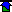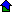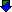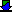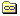Bob UK
Unregistered guest
Posted From: 94.197.122.80
 Posted on Wednesday, 31 December, 2014 - 06:43: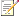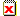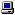Always remember this learn it.

A circuit that has 1 volt and 1 amp is 1 watt and has a resistance of 1 ohm and a capacitance of 1 farad and a power consumption of 1 coloumb and the ohms is inversely proportional

For car stuff ignore coloumbs and farads. Coloumbs is watts per second.

This leaves watts amps ohms and volts.

ohms law,
volts = amps x ohms.

Watts law.
Watts = volts x amps.

Therefore.
Watts = amps x amps x ohms.

Or watts =amps squared x ohms.

Eg. 12v x 6 amps = 72 watts.

6amps x 6amps x 2 ohms =72w.

6amps x 2 ohms = 12v.

The laws apply dead on the money in both theory and practice.

The water analogy.

volts is Water pressure.

Amps is the amount flowing.

Ohms or resistance is the size of the hole Water is flowing though. The smaller the hole the larger the resistance to flow.

Watts. Imagine this is an impulse water wheel.

To satisfy your self, get a indicator bulb and connect to 12v battery. Then using a digital meter. Measure battery voltage with bulb lit. Then turn off measure the ohms of the bulb. Then connect ammeter in series with bulb on.

Amps x volts equal watts. When calculated it won't be exactly 21w. Because the wire has resistance, the volts wasn't exactly 12 and bulb makers tolerances.

Notice that watts which is power is measured indirectly.

AC systems. Apart from the alternator stator and audio equipment cars are DC.

The laws apply to ac as well.

These laws do not show what size wire to use. The size of the conductor is listed in tables. The max amperage is used to determine the size. The insulation is chosen to resist the voltage pressure high voltage pressure thick insulation.

All conductors have resistance. All insulators have resistance. The difference is that some materials are better at conducting than resisting, and viva versus. Some materials are half way and are called semi conductors, or if you like semi insulators.

Audio stuff. The output to speakers is AC. AC current at high frequency 20Hz to 20,000Hz. AC because is is changing polarity tends to sit on the surface of the wire leaving the middle at lower volts. It doesn't get time to sink in like constant DC. This means that speaker wiring should be the correct stuff. Not necessarily expensive just the correct stuff.
Impedance is a measure of resistance with an AC phase angle attached. Because DC has no phase the angle is zero so DC stuff uses resistance. Impedance and resistance use ohms as a unit. Impedance is denoted by Z and resistance by R.

Note when doing electrical maths for cars, remember that the voltage is not 12 it is 12 to 14.4. Bulbs although marked 12 are actually rated at 14.

Phase angle because ac is usually made by rotating machinery where 1 phase is 360 or one turn and 3 phase is 120 or a third of a turn.

Coloumbs or watts per second is like kilowatt hour only 3600000 smaller.

Farads are the size of the tank whether is empty or full. My petrol tank is 23 gallons. It each gallon was a farad then I could say it has a capacitance of 23 farads.
Capacitor.( Condenser is the same)

Wikipedia is a good place to start.

(Message approved by david_gore)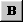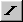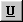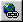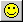-Color- Black Red Green Blue Yellow Purple Orange Cyan Gray White -Font- Arial Courier Symbol Times Verdana -Size- Smallest (-2) Small (-1) Normal Large (+1) Largest (+2)